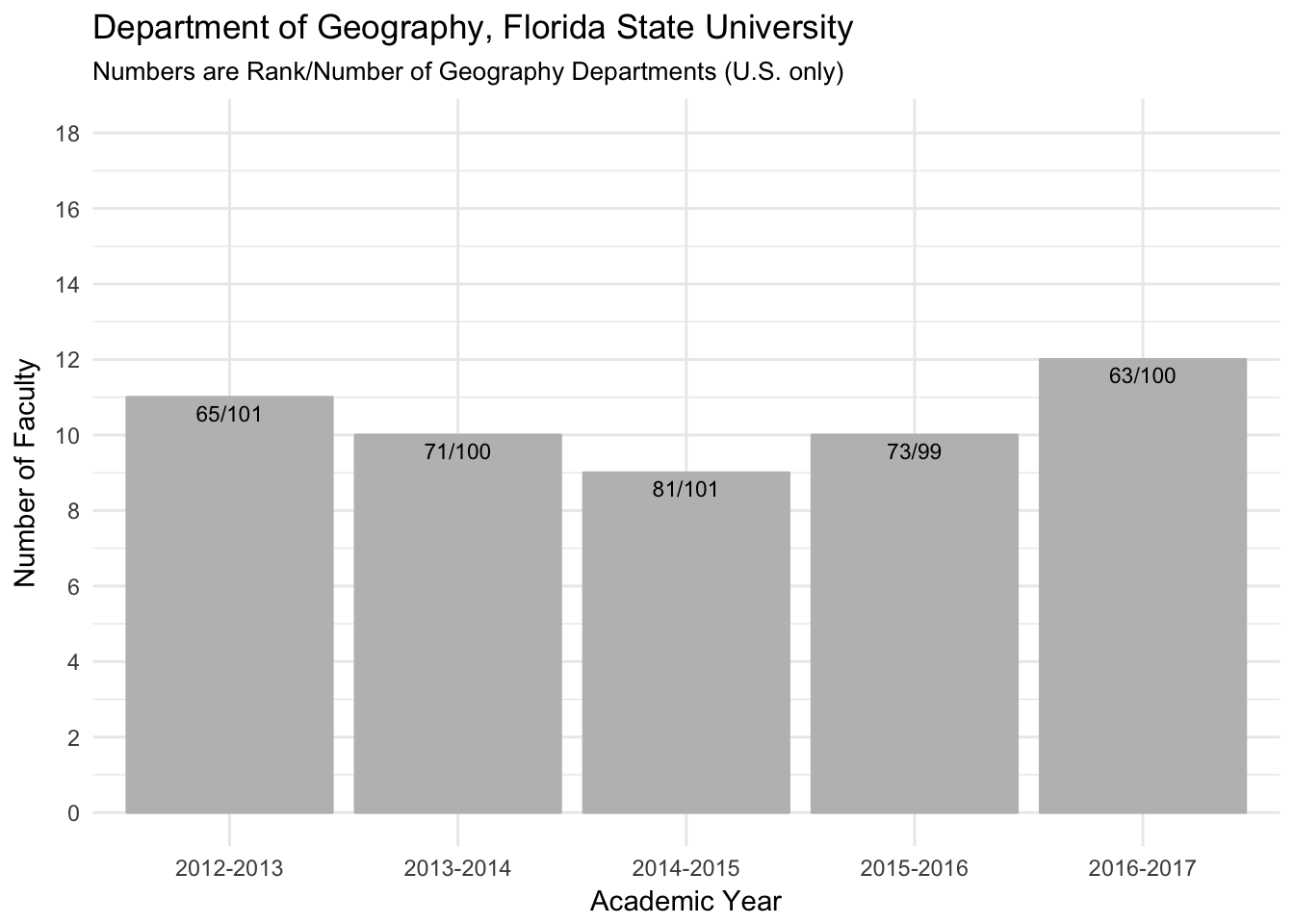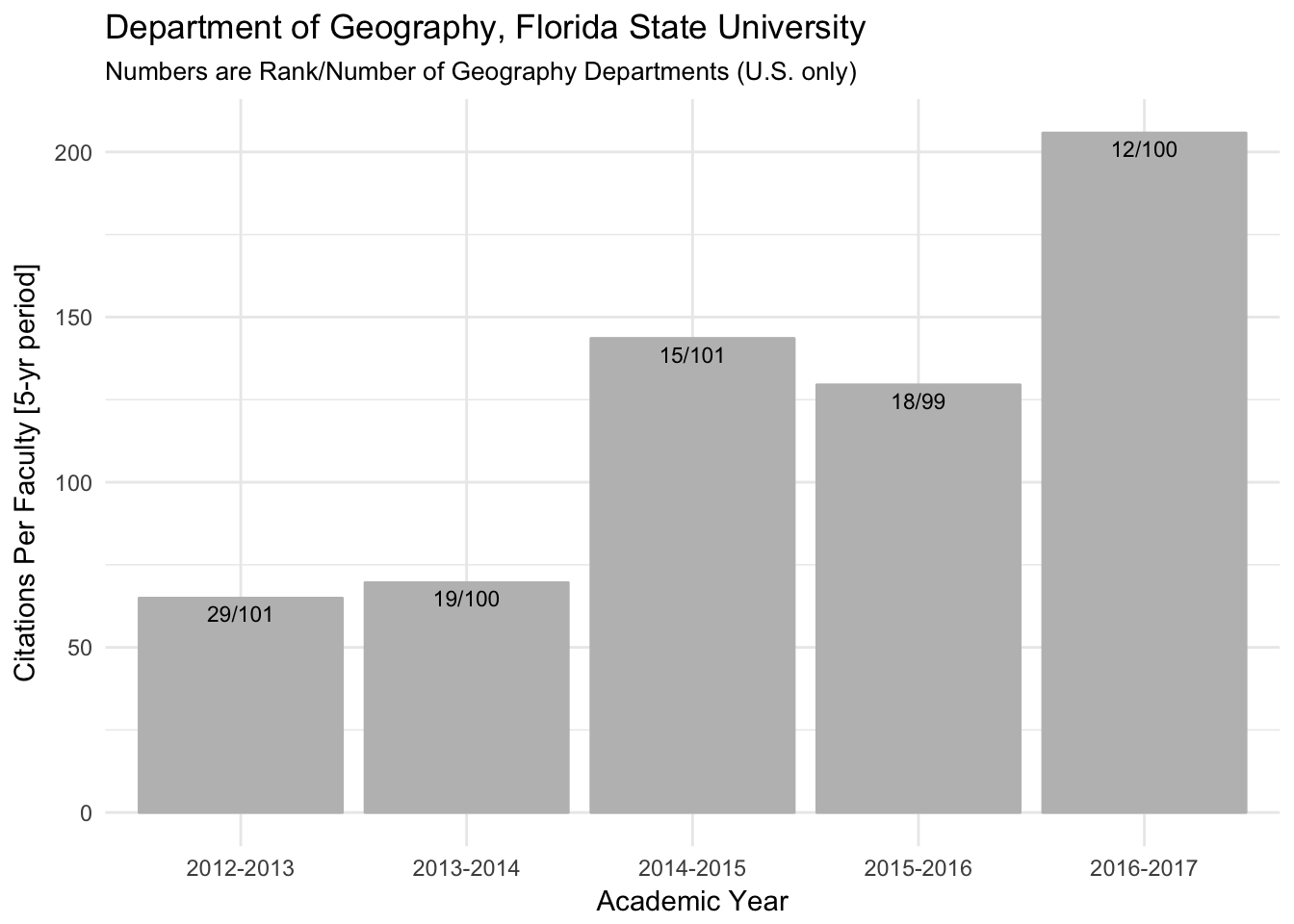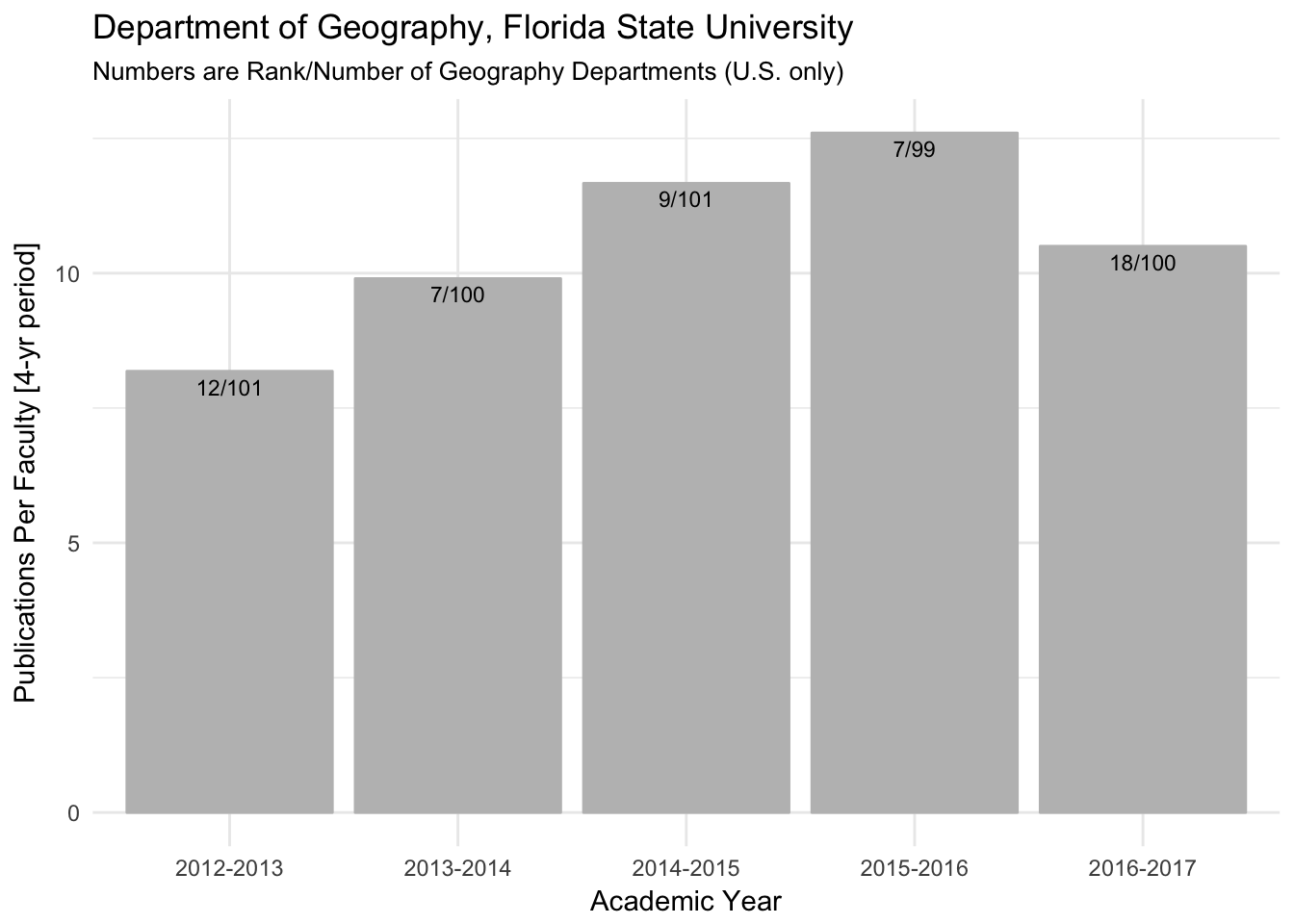# Five Year Trends

Over the holidays I took a look at how we’ve been doing as a department over the past five years. There are many ways to do this but I thought it would be instructive to quantify our productivity relative to the 100 or so geography departments in the country.

The central message is that, although we are a relatively small department, we are writing lots of papers and these papers are receiving good attention. Happy New Year and keep up the great work.

The procedure I used and the output are explained through the following R code.

Download `FullData.csv` from https://portal2.academicanalytics.com/Account/Login?ReturnUrl=%2F

User name: `JElsner@73` Password (case sensitive): `xxxxxx`

``library(tidyverse)``
``## ── Attaching packages ────────────────────────────────── tidyverse 1.2.1 ──``
``````## ✔ ggplot2 2.2.1     ✔ purrr   0.2.4
## ✔ tibble  1.3.4     ✔ dplyr   0.7.4
## ✔ tidyr   0.7.2     ✔ stringr 1.2.0
## ✔ readr   1.1.1     ✔ forcats 0.2.0``````
``````## ── Conflicts ───────────────────────────────────── tidyverse_conflicts() ──

Input data from latest release.

``df2016 = read.csv(file = "FullData2016.csv")``

AAD2016.01.814 Release Date: 11/21/2017 Weighting: AAD2016 Faculty: Academic Year 2016 – 2017 Journal Articles: 2013 – 2016 Awards: No Limit Books: 2007 – 2016 Citations: 2012 – 2016 Conference Proceedings: 2013 – 2016 Grants: 2012 – 2016

Sort queries for last academic year.

``````results = df2016 %>%
select(institutionname, numfac, totpubs, totcits) %>%
mutate(pubspfac = totpubs/numfac,
citspfac = totcits/numfac,
rank = rank(desc(citspfac), ties.method = "min")) %>%
arrange(rank)
results\$rank[results\$institutionname == "Florida State University"]``````
``##  12``

Input data from earlier years.

``````df2015 = read.csv(file = "FullData2015.csv")

df2016\$Year = rep("2016-2017", dim(df2016))
df2015\$Year = rep("2015-2016", dim(df2015))
df2014\$Year = rep("2014-2015", dim(df2014))
df2013\$Year = rep("2013-2014", dim(df2013))
df2012\$Year = rep("2012-2013", dim(df2012))

dfAll = rbind(df2016, df2015, df2014, df2013, df2012)``````

Five-year trends

``````results = dfAll %>%
select(Year, institutionname, numfac, totpubs, totcits, ranknumfac) %>%
group_by(Year) %>%
mutate(nInst = n(),
pubspfac = totpubs/numfac,
citspfac = totcits/numfac,
rankCits = rank(desc(citspfac), ties.method = "min"),
rankPubs = rank(desc(pubspfac), ties.method = "min")) %>%
filter(institutionname == "Florida State University") %>%
select(Year, ranknumfac, rankCits, rankPubs, numfac, citspfac, pubspfac, nInst)``````

Graphs:

Number of faculty

``````ggplot(results, aes(x = Year, y = numfac)) +
geom_bar(stat = "identity", fill = "gray", color = "gray") +
geom_text(aes(label = paste0(ranknumfac, "/", nInst)), vjust = 1.5, size = 3) +
scale_y_continuous(breaks = seq(0, 18, 2), limits = c(0, 18)) +
ylab("Number of Faculty") +
ggtitle("Department of Geography, Florida State University", subtitle = "Numbers are Rank/Number of Geography Departments (U.S. only)") +
theme_minimal()``````Citations per faculty

``````ggplot(results, aes(x = Year, y = citspfac)) +
geom_bar(stat = "identity", fill = "gray", color = "gray") +
geom_text(aes(label = paste0(rankCits, "/", nInst)), vjust = 1.5, size = 3) +
ylab("Citations Per Faculty [5-yr period]") +
ggtitle("Department of Geography, Florida State University", subtitle = "Numbers are Rank/Number of Geography Departments (U.S. only)") +
theme_minimal()``````Publications per faculty

``````ggplot(results, aes(x = Year, y = pubspfac)) +
geom_bar(stat = "identity", fill = "gray", color = "gray") +
geom_text(aes(label = paste0(rankPubs, "/", nInst)), vjust = 1.5, size = 3) +